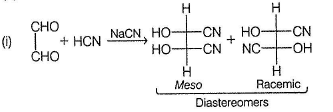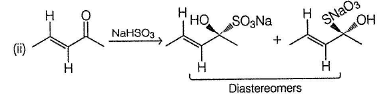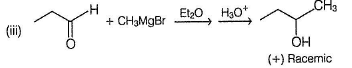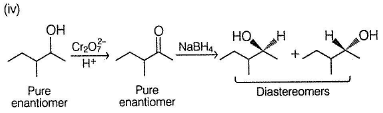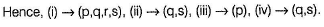Courses

# Test: Physical Properties And General Reactions

## 24 Questions MCQ Test Chemistry Class 12 | Test: Physical Properties And General Reactions

Description
This mock test of Test: Physical Properties And General Reactions for Class 12 helps you for every Class 12 entrance exam. This contains 24 Multiple Choice Questions for Class 12 Test: Physical Properties And General Reactions (mcq) to study with solutions a complete question bank. The solved questions answers in this Test: Physical Properties And General Reactions quiz give you a good mix of easy questions and tough questions. Class 12 students definitely take this Test: Physical Properties And General Reactions exercise for a better result in the exam. You can find other Test: Physical Properties And General Reactions extra questions, long questions & short questions for Class 12 on EduRev as well by searching above.
QUESTION: 1

### Only One Option Correct Type Direction (Q, Nos. 1-9) This section contains 9 multiple choice questions. Each question has four choices (a), (b), (c) and (d), out of which ONLY ONE is correct. Q.  Which of the following will give a racemic mixture on reduction with NaBH4 followed by acid work-up?

Solution:

NaBH4 brings about reduction of carbonyls by hydrid e transfer mechanism at planar sp2 carbon. Hence, if a chiral carbon is generated, racemic mixture is always produced.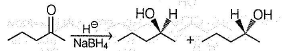QUESTION: 2

### What would be the major product in the following reaction?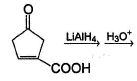Solution:

LiAIH4 reduces both carbonyls and carboxylic groups but does not reduce olefinic bond.

QUESTION: 3

### Which of the following on reaction with excess of NaHSO3 in aqueous solution will give mixture of salts which can be separated into two fractions by fractional crystallisation?

Solution: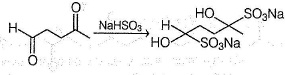In the above reaction, four stereoisomers, two pairs of enantiomers are formed. Each member of a pair of enantiomer is diastereomer of each member of other pair of enantiomer. Hence, fractional crystallisation would give two fractions, each containing racemic mixture.

QUESTION: 4

Which is the most suitable reagent for the following transformation?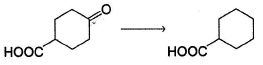Solution:

Clemmensen reduction is suitable for reductio n of carbonyls containing additional acidic functional group.

QUESTION: 5

Which is the most suitable reagent for the following transformation?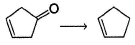Solution:

Wolff-Kishner reduction is suitable for reduction of carbonyls containing olefinic bonds. If Clemmensen reduction is done, HCI also attacks olefinic bonds.

QUESTION: 6

The reagent which can best bring about the following transformation is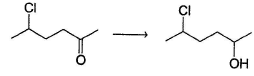Solution:

With aluminium isopropoxide (MPV reduction), carbonyls are selectively reduced to alcohols leaving other groups intact.

QUESTION: 7

The most probable product of the following reaction is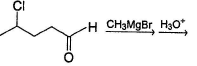Solution: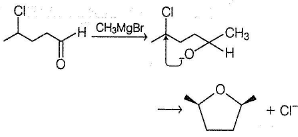If possible, intramolecular reaction is preferred over intermolecular reaction.

QUESTION: 8

Consider the following reaction,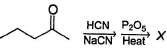Q.

The most likely organic product X is

Solution: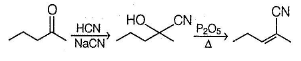QUESTION: 9

Consider the following reaction,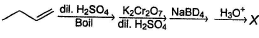Q.

The most likely organic product X is

Solution: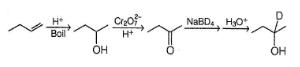*Multiple options can be correct
QUESTION: 10

One or More than One Options Correct Type

Direction (Q. Nos. 10-13) This section contains 4 multiple choice questions. Each question has four choices (a), (b), (c) and (d), out of which ONE or MORE THAN ONE are correct.

Consider the following reaction,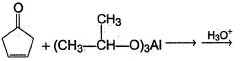Q.

The organic product(s) formed above is/are

Solution:

In MPV reduction, carbonyl is reduced to alcohol while isopropoxide fraction of reducing agent is oxidised to acetone.

*Multiple options can be correct
QUESTION: 11

Consider the reaction given below,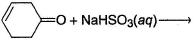Q.

The correct observation regarding the above reaction is/are

Solution:

Aldehydes and ketones form bisulp hite salt with NaHSO3 which is insoluble in concentrated NaHSO3 solution due to common ion effect. Hence, if NaHSO3 is in limited quantity, precipitation may not take place.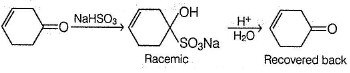*Multiple options can be correct
QUESTION: 12

Consider the reaction given below,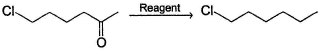Q.

The correct deduction(s) regarding the above reagent is/are

Solution:

Reducing agent (Zn(Hg)-HCI) in Clemmensen reduction does not affect the halide group. If W olff-Kishner (N2H4 - OH-) reduction is done, OH- may bring about SN2 and E2 reaction with halide group.

*Multiple options can be correct
QUESTION: 13

Consider the following reaction,Q.

The correct deduction(s) regarding mechanism of the above reaction is/are

Solution:

H- (hydride) ion undergoes nu cle ophilic ad dition at sp2 carbon of carbonyls. Aldehydes are more reactive than ketones due to less steric hindrance in former case. Since, nucleophilic attack of hydride ion occur at planar carbonyl carbon, there is equal probability of attack from both side of plane giving racemic mixture if chiral carbon is generated.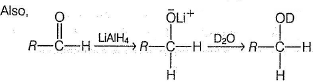QUESTION: 14

Comprehension Type

Direction (Q. Nos. 14-16) This section contains a paragraph, describing theory, experiments, data, etc. Three questions related to the paragraph have been given. Each question has only one correct answer among the four given options (a), (b), (c) and (d).

Passage

An organic compound A (C7H16O) shows both enantiomerism and diastereomerism. Treatment of a pure enantiomer of A with Na2CrO4 /Dil. H2SO4 gives B (C7H14O) - Also A on dehydration with concentrated H2SO4 gives a single alkene C (C7H14). Ozonolysis of C followed by work-up with Zn-H2O gives D (C5H10O) as one of the product which gives racemic mixture on reduction with NaBH4.

Q.

The structure of A satisfying the above criteria is

Solution:

Since, A is completely saturated, it must contain more than one chiral carbon atoms in order to show both enantiomerism and diastereomerism. Also, C on ozonolysis gives D (C5H10O) as one product, other product must be CH3SHO. Hence, C must be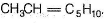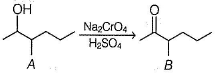QUESTION: 15

An organic compound A (C7H16O) shows both enantiomerism and diastereomerism. Treatment of a pure enantiomer of A with Na2CrO4 /Dil. H2SO4 gives B (C7H14O) - Also A on dehydration with concentrated H2SO4 gives a single alkene C (C7H14). Ozonolysis of C followed by work-up with Zn-H2O gives D (C5H10O) as one of the product which gives racemic mixture on reduction with NaBH4.

Q.

If B is reduced with LiAIH4 followed by acid hydrolysis will give

Solution:

Since, A is completely saturated, it must contain more than one chiral carbon atoms in order to show both enantiomerism and diastereomerism. Also, C on ozonolysis gives D (C5H10O) as one product, other product must be CH3SHO. Hence, C must beB is enantiomeric. If a pure enantiomer of B is reduced with LiAIH4, pair of diastereomers would be formed.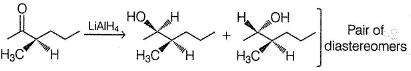QUESTION: 16

An organic compound A (C7H16O) shows both enantiomerism and diastereomerism. Treatment of a pure enantiomer of A with Na2CrO4 /Dil. H2SO4 gives B (C7H14O) - Also A on dehydration with concentrated H2SO4 gives a single alkene C (C7H14). Ozonolysis of C followed by work-up with Zn-H2O gives D (C5H10O) as one of the product which gives racemic mixture on reduction with NaBH4.

Q.

The correct statement regarding the compound D is

Solution:

Since, A is completely saturated, it must contain more than one chiral carbon atoms in order to show both enantiomerism and diastereomerism. Also, C on ozonolysis gives D (C5H10O) as one product, other product must be CH3SHO. Hence, C must be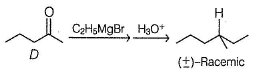*Answer can only contain numeric values
QUESTION: 17

One integer Value Correct Type

Direction (Q. Nos. 17-22) This section contains 6 questions. When worked out will result in an integer from 0 to 9 (both inclusive).

Q.

If all the aldehyde isomers of C5H10O is independently treated with HCN/NaCN solution, how many of them will give racemic mixture of cyanohydrin?

Solution: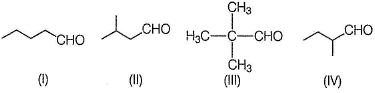IV is enantiomeric. Its pure enantiomer, with HCN/NaCN, would produce pair of diastereomers.

*Answer can only contain numeric values
QUESTION: 18

If one molecule of trioxane is heated, how many molecules of formaldehyde would be formed?

Solution: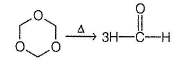*Answer can only contain numeric values
QUESTION: 19

A mixture containing all isomeric cyclohexanedione is treated with excess of NaHSO3 solution. How many different disulphite salts are formed?

Solution: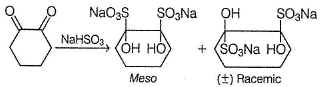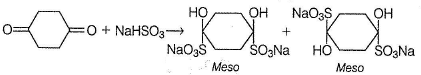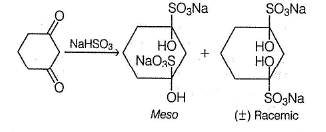*Answer can only contain numeric values
QUESTION: 20

Consider the following reaction,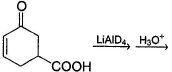Q

How many deuterium would be incorporated in the final product?

Solution: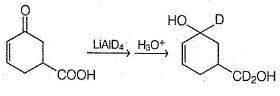*Answer can only contain numeric values
QUESTION: 21

If glyoxal glycol is treated with a mixture of CH3MgBr and C2H5MgBr in diethyl ether followed by acid hydrolysis, how many different diols would be formed, which are simultaneously optically active?

Solution: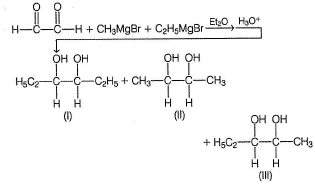One pair of enantiomers for each (I) and (II) while two pairs of enantiomers for (III).

*Answer can only contain numeric values
QUESTION: 22

Consider the reaction given below,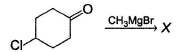Q.

X is formed in which a new ring is formed. How many atoms are present in this new ring?

Solution: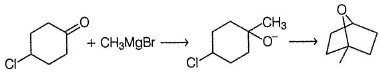QUESTION: 23

Matching List Type

Direction (Q. Nos. 23 and 24) Choices for the correct combination o f elements from Column I and Column II are given as options (a), (b), (c) and (d), out of which one is correct.

Q.

Match the reactants from Column I with the reagents and expected outcomes from Column II. Mark the correct option form the codes given below.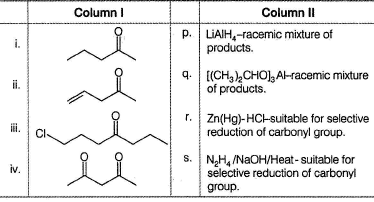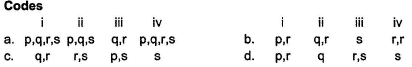Solution:

(i) It has a carbonyl carbon that turns chiral on reduction with hydrides. Hence, with LiAIH4 or aluminium isopropoxide, gives racemic mixture. Also, it has no other functional groups, either Clemmensen reduction or Wolff-Kishner reduction can be used.
(ii) It has an olefinic double bond, Clemmensen reduction would not be suitable for selective reduction of carbonyl group.
(iii) LiAlH4 also reduces primary halide but aluminium isopropoxide does not. Wolff-Kishner reduction would not be suitable because HO- reacts with halide group (EN2 or E2).
(iv) Same reasons as with (i).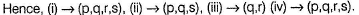QUESTION: 24

Match the reactions from Column I with the properties of products from Column II. Mark the correct option form the codes given below.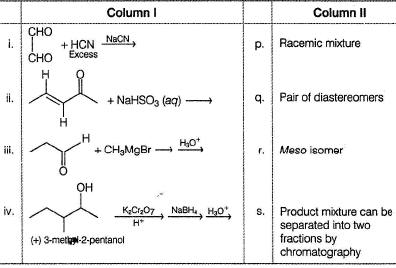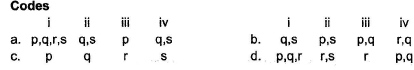Solution: